# Ackermann Function

• Last Updated : 11 Jun, 2021

In computability theory, the Ackermann function, named after Wilhelm Ackermann, is one of the simplest and earliest-discovered examples of a total computable function that is not primitive recursive. All primitive recursive functions are total and computable, but the Ackermann function illustrates that not all total computable functions are primitive recursive. Refer this for more.
It’s a function with two arguments each of which can be assigned any non-negative integer.
Ackermann function is defined as: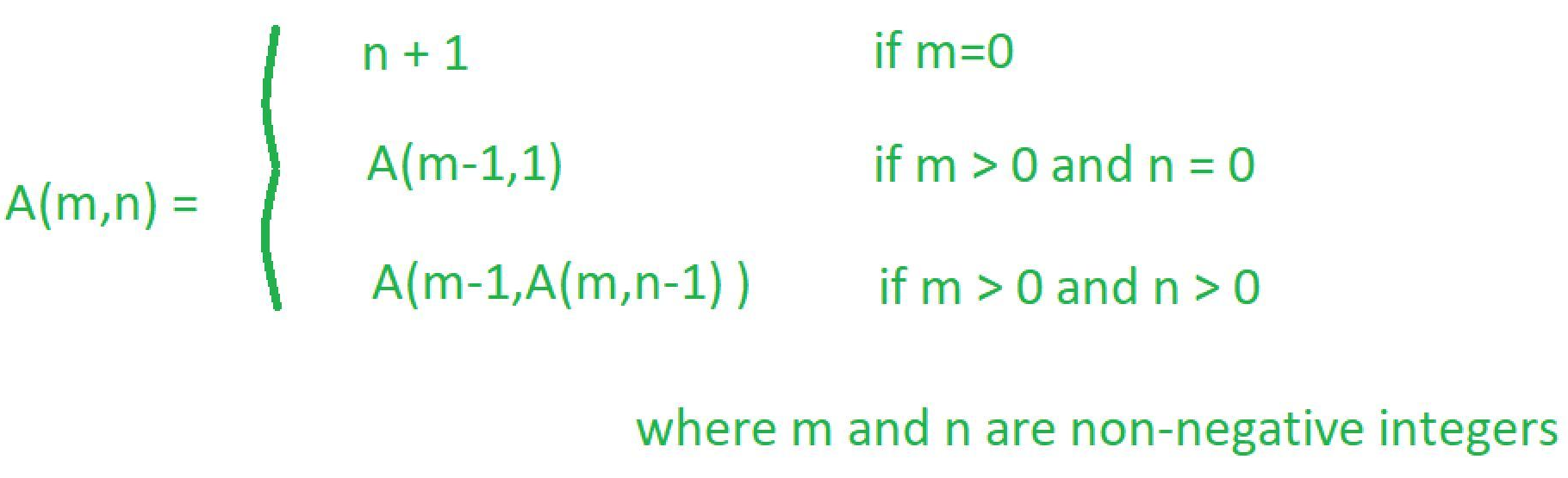Ackermann algorithm:

```Ackermann(m, n)
{next and goal are arrays indexed from 0 to m, initialized so that next[O]
through next[m] are 0, goal[O] through goal[m - l] are 1, and goal[m] is -1}
repeat
value <-- next[O] + 1
transferring <-- true
current <-- O
while transferring do begin
if next[current] = goal[current] then goal[current] <-- value
else transferring <-- false
next[current] <-- next[current]+l
current <-- current + 1
end while
until next[m] = n + 1
return value {the value of A(m, n)}
end Ackermann ```

Here’s the explanation of the given Algorithm:
Let me explain the algorithm by taking the example A(1, 2) where m = 1 and n = 2
So according to the algorithm initially the value of next, goal, value and current are: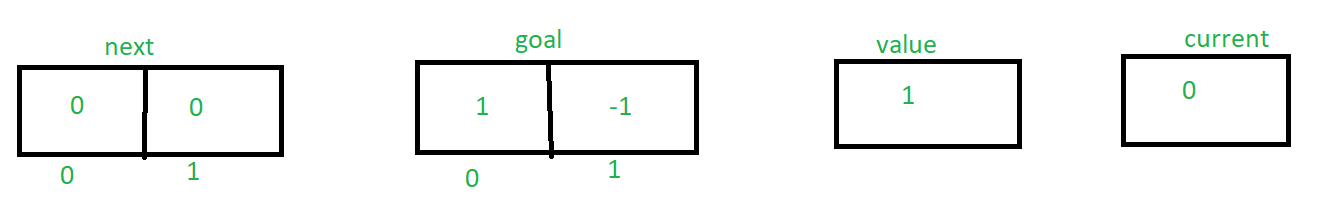Though next[current] != goal[current], so else statement will execute and transferring become false.
So now, the value of next, goal, value and current are: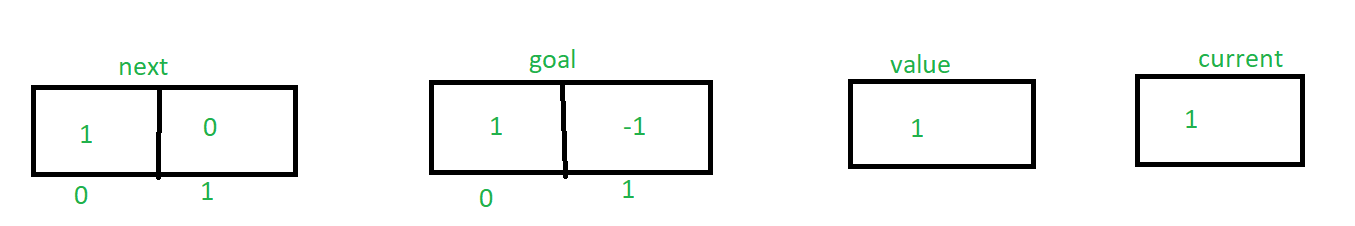Similarly by tracing the algorithm until next[m] = 3 the value of next, goal, value and current are changing accordingly. Here’s the explanation how the values are changing,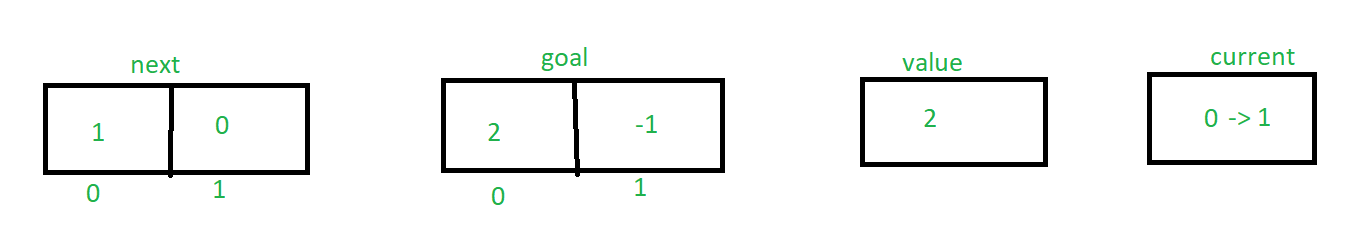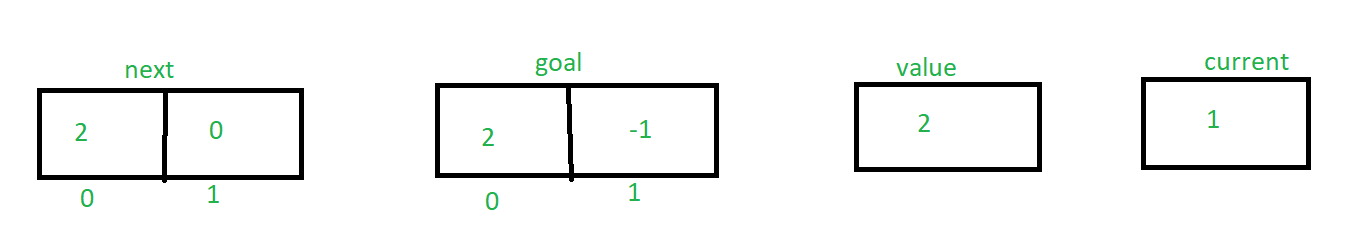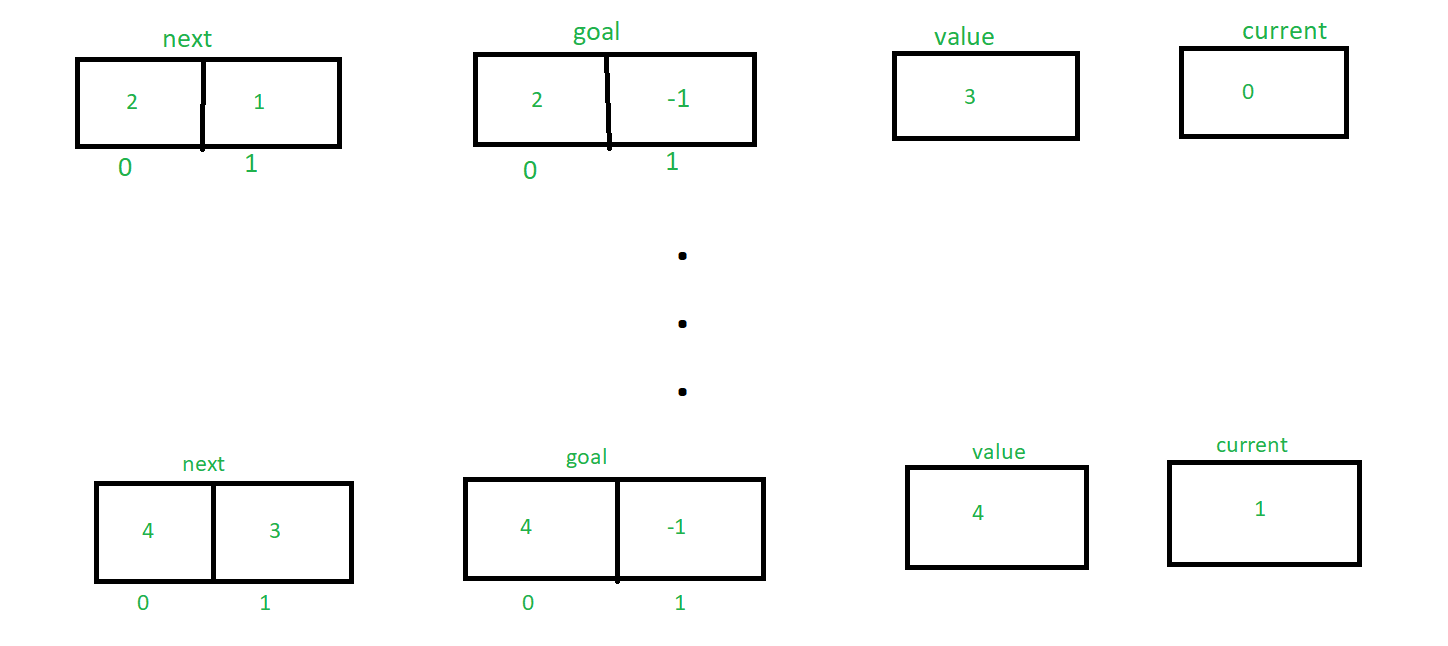Finally returning the value e.g 4
Analysis of this algorithm:

• The time complexity of this algorithm is: O(mA(m, n)) to compute A(m, n)

• The space complexity of this algorithm is: O(m) to compute A(m, n)

Let’s understand the definition by solving a problem!

Solve A(1, 2)?
Given problem is A(1, 2)
Here m = 1, n = 2 e.g m > 0 and n > 0
Hence applying third condition of Ackermann function
A(1, 2) = A(0, A(1, 1)) ———- (1)
Now, Let’s find A(1, 1) by applying third condition of Ackermann function
A(1, 1) = A(0, A(1, 0)) ———- (2)
Now, Let’s find A(1, 0) by applying second condition of Ackermann function
A(1, 0) = A(0, 1) ———- (3)
Now, Let’s find A(0, 1) by applying first condition of Ackermann function
A(0, 1) = 1 + 1 = 2
Now put this value in equation 3
Hence A(1, 0) = 2
Now put this value in equation 2
A(1, 1) = A(0, 2) ———- (4)
Now, Let’s find A(0, 2) by applying first condition of Ackermann function
A(0, 2) = 2 + 1 = 3
Now put this value in equation 4
Hence A(1, 1) = 3
Now put this value in equation 1
A(1, 2) = A(0, 3) ———- (5)
Now, Let’s find A(0, 3) by applying first condition of Ackermann function
A(0, 3) = 3 + 1 = 4
Now put this value in equation 5
Hence A(1, 2) = 4
So, A (1, 2) = 4

Let’s solve another two questions on this by yourself!
Question: Solve A(2, 1)?
Question: Solve A(2, 2)?

Here is the simplest c and python recursion function code for generating Ackermann function

## C++

 `// C++ program to illustrate Ackermann function``#include ``using` `namespace` `std;` `int` `ack(``int` `m, ``int` `n)``{``    ``if` `(m == 0){``        ``return` `n + 1;``    ``}``    ``else` `if``((m > 0) && (n == 0)){``        ``return` `ack(m - 1, 1);``    ``}``    ``else` `if``((m > 0) && (n > 0)){``        ``return` `ack(m - 1, ack(m, n - 1));``    ``}``}` `// Driver code``int` `main()``{``    ``int` `A;``    ``A = ack(1, 2);``    ``cout << A << endl;``    ``return` `0;``}` `// This code is contributed by SHUBHAMSINGH10`

## C

 `// C program to illustrate Ackermann function` `#include ``int` `ack(``int` `m, ``int` `n)``{``    ``if` `(m == 0){``        ``return` `n+1;``    ``}``    ``else` `if``((m > 0) && (n == 0)){``        ``return` `ack(m-1, 1);``    ``}``    ``else` `if``((m > 0) && (n > 0)){``        ``return` `ack(m-1, ack(m, n-1));``    ``}``}` `int` `main(){``    ``int` `A;``    ``A = ack(1, 2);``    ``printf``(``"%d"``, A);``    ``return` `0;``}` `// This code is contributed by Amiya Rout`

## Java

 `// Java program to illustrate Ackermann function` `class` `GFG``{` `    ``static` `int` `ack(``int` `m, ``int` `n)``    ``{``        ``if` `(m == ``0``)``        ``{``            ``return` `n + ``1``;``        ``}``        ``else` `if``((m > ``0``) && (n == ``0``))``        ``{``            ``return` `ack(m - ``1``, ``1``);``        ``}``        ``else` `if``((m > ``0``) && (n > ``0``))``        ``{``            ``return` `ack(m - ``1``, ack(m, n - ``1``));``        ``}``else``        ``return` `n + ``1``;``    ``}` `    ``// Driver code``    ``public` `static` `void` `main(String args[])``    ``{``        ``System.out.println(ack(``1``, ``2``));``    ``}``}` `// This code is contributed by AnkitRai01`

## Python3

 `# Python program to illustrate Ackermann function` `def` `A(m, n, s ``=``"% s"``):``    ``print``(s ``%` `(``"A(% d, % d)"` `%` `(m, n)))``    ``if` `m ``=``=` `0``:``        ``return` `n ``+` `1``    ``if` `n ``=``=` `0``:``        ``return` `A(m ``-` `1``, ``1``, s)``    ``n2 ``=` `A(m, n ``-` `1``, s ``%` `(``"A(% d, %% s)"` `%` `(m ``-` `1``)))``    ``return` `A(m ``-` `1``, n2, s)` `print``(A(``1``, ``2``))` `# This code is contributed by Amiya Rout`

## C#

 `// C# program to illustrate Ackermann function``using` `System;` `class` `GFG``{` `    ``static` `int` `ack(``int` `m, ``int` `n)``    ``{``        ``if` `(m == 0)``        ``{``            ``return` `n + 1;``        ``}``        ``else` `if``((m > 0) && (n == 0))``        ``{``            ``return` `ack(m - 1, 1);``        ``}``        ``else` `if``((m > 0) && (n > 0))``        ``{``            ``return` `ack(m - 1, ack(m, n - 1));``        ``}``else``        ``return` `n + 1;``    ``}` `    ``// Driver code``    ``public` `static` `void` `Main()``    ``{``        ` `        ``Console.WriteLine(ack(1, 2));``    ``}``}` `// This code is contributed by mohit kumar 29`

## Javascript

 ``

If you still wish to visualize how this result is arrived at, you can take a look at this page, which animates the calculation of every recursion step.

My Personal Notes arrow_drop_up